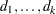The ARIMA Procedure
 OUTLIER Statement
OUTLIER options ;

The OUTLIER statement can be used to detect shifts in the level of the response series that are not accounted for by the previously estimated model. An ESTIMATE statement must precede the OUTLIER statement. The following options are used in the OUTLIER statement:

TYPE=SHIFT
TYPE=TEMP ()
TYPE=(<ADDITIVE><SHIFT> <TEMP () )>

specifies the types of level shifts to search for. The default is TYPE=(ADDITIVE SHIFT), which requests searching for additive outliers and permanent level shifts. The option
TEMP() requests searching for temporary changes in the level of durations. These options can also be abbreviated as AO, LS, and TC.

ALPHA=significance-level

specifies the significance level for tests in the OUTLIER statement. The default is 0.05.

SIGMA=ROBUST | MSE

specifies the type of error variance estimate to use in the statistical tests performed during the outlier detection. SIGMA=MSE corresponds to the usual mean squared error (MSE) estimate, and SIGMA=ROBUST corresponds to a robust estimate of the error variance. The default is SIGMA=ROBUST.

MAXNUM=number

limits the number of outliers to search. The default is MAXNUM=5.

MAXPCT=number

limits the number of outliers to search for according to a percentage of the series length. The default is MAXPCT=2. When both the MAXNUM= and MAXPCT= options are specified, the minimum of the two search numbers is used.

ID=Date-Time ID variable

specifies a SAS date, time, or datetime identification variable to label the detected outliers. This variable must be present in the input data set.

The following examples illustrate a few possibilities for the OUTLIER statement.

The most basic usage, shown as follows, sets all the options to their default values.

```      outlier;
```

That is, it is equivalent to

```      outlier type=(ao ls) alpha=0.05 sigma=robust maxnum=5 maxpct=2;
```

The following statement requests a search for permanent level shifts and for temporary level changes of durations 6 and 12. The search is limited to at most three changes and the significance level of the underlying tests is 0.001. MSE is used as the estimate of error variance. It also requests labeling of the detected shifts using an ID variable date.

```      outlier type=(ls tc(6 12)) alpha=0.001 sigma=mse maxnum=3 ID=date;
```Previous Page | Next Page | Top of Page